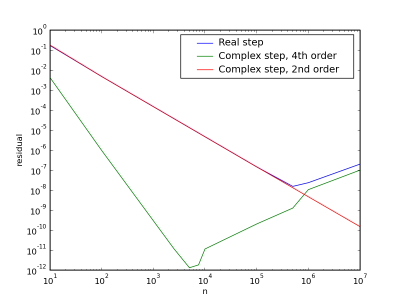## Tuesday, December 2, 2008

### Complex Step: Subtractive Cancellation

We showed in an earlier post that approximating first derivatives with complex steps leads to a second order accurate formula that avoids the subtractive cancellation associated with a normal finite difference.

Following the simple convergence study for second derivative approximations we did for cos(). We'll look at the convergence of some first derivative approximation formulas.

The simple complex step approximation is given byThe standard second order finite difference is given byThe 4th order combined complex and real step approximation isNotice that this formula is not able to avoid subtractive cancellation the way the second order approximation does, but we get a fourth order accurate approximation on a compact stencil.

The convergence of these three formulas for approximating the derivative of cosine is shown in the figure below.The 4th order scheme runs into subtractive cancellation earlier than the standard 2nd order scheme, but it remains more accurate over the examined range of resolution. The complex step approximation continues to converge as the step size decreases even after the other two formulas diverge.

So for doing sensitivity analysis the second order complex step formula should be used, because only one step needs to be taken, and arbitrarily high precision can be achieved. If you need to approximate a derivative over a region, then the higher order method will pay-off because you can get better accuracy with fewer points.

#### 1 comment:

1.This method might get implemented in Maxima for calculating very accurate numerical derivatives.